# Machine learning¶

## Calibration and selection¶

How to tune a machine learning model and select the best one.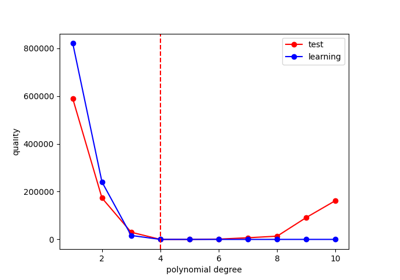Calibration of a polynomial regression

Calibration of a polynomial regression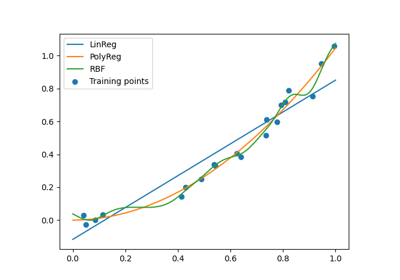Machine learning algorithm selection example

Machine learning algorithm selection example

## Classification¶

How to create a classification model.Classification API

Classification APIK nearest neighbors classification

K nearest neighbors classificationRandom forest classification

Random forest classification

## Clustering¶

How to create a clustering model.API

APIGaussian Mixtures

Gaussian MixturesK-means

K-means

## Dimension reduction¶

How to reduce the dimension of a high-dimensional variable.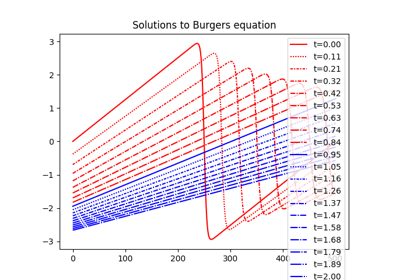KL-SVD on Burgers equation

KL-SVD on Burgers equation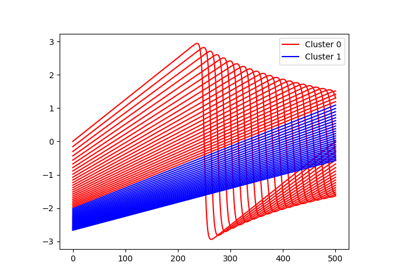Mixture of experts with PCA on Burgers dataset

Mixture of experts with PCA on Burgers datasetPCA on Burgers equation

PCA on Burgers equation

## Quality¶

How to assess the quality of a machine learning model.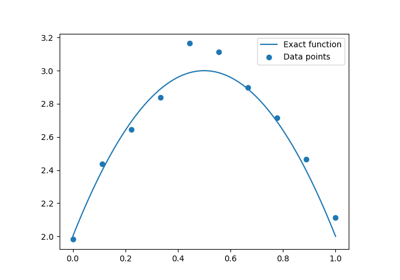MSE example - test-train split

MSE example - test-train split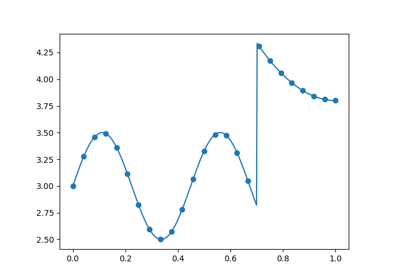Quality measure for surrogate model comparison

Quality measure for surrogate model comparison

## Regression¶

How to create a regression model.API

APIGP regression

GP regressionLinear regression

Linear regression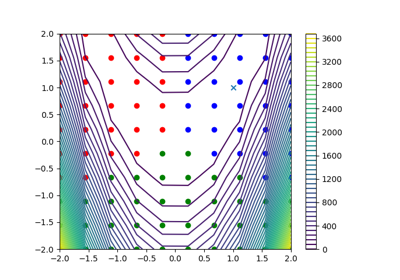Mixture of experts

Mixture of expertsPCE regression

PCE regressionPolynomial regression

Polynomial regressionRBF regression

RBF regressionRandom forest regression

Random forest regression## Data transformation¶

How to transform the data before training.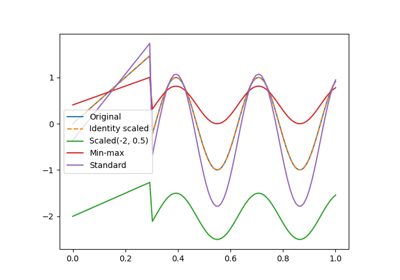Scaler example

Scaler example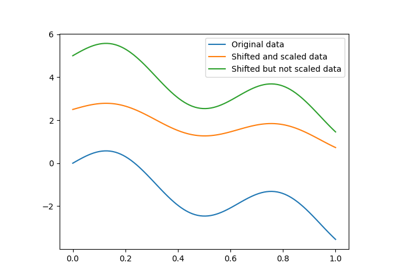Transformer pipeline example

Transformer pipeline example

Gallery generated by Sphinx-Gallery